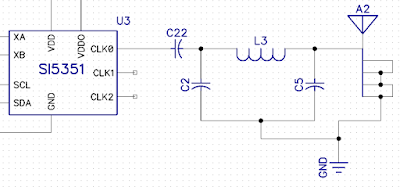## Saturday, February 13, 2016

### WSPR tracker output filters

I'm in the midst of designing a small WSPR tracker based on SMD technology for use with Pico Balloons.  I hope to attempt a circumnavigation with my design and home built envelope.

My tracker will be using a Silicon labs Si5351 CMOS clock generator.  Per the datasheet, the clock generator is spec'd to an 50 ohm output impedance.   Note, that there are different Si5351 parts. The "-B" parts seem to be the ones you can find now, and they have 50 ohm termination.  The earlier non-"-B" parts, were rated at 85 ohm.

Looking at the output of an Adafruit Si5351 protoboard on a spectrum analyzer, the output signal would definitely benefit from some filtering.There are some sizable harmonics.

First pass at a filter plan

I'm new to RF design, but as I understand it, I should probably have a DC Coupling Capacitor as well as a filter network.  I'm going to begin with a simple Pi Lowpass filter and see how that goes.In this design, C22 is the coupling cap, and the Pi filter is comprised of C2, C5 and L3.

Coupling Cap

The target output frequency is on 30m, at about 10.1 Mhz.  I found the following gem on the interwebz, so it must be true:
You generally want the capacitor reactance (impedance) to be much less than the circuit impedance.
The reactance of a capacitor is Xc = 1/(2*pi*f*C) where f is the frequency of operation.
Thus the higher the frequency and the larger the capacitor, the smaller the reactance.
Thus for a typical 50Ω circuit impedance, you probably should have the capacitive impedance no more that about 1% of 50Ω or 0.5Ω.
So, for example, at 2.4GHz frequency, the capacitor should be no smaller than C = 1/(2*pi*2.4GHz*0.5Ω) = 132pF.
Substituting in 10.1Mhz into the formula, I get a value of 31.5nf.

Filter values

I found a Chebyshev Pi LC Low Pass Filter Calculator on the web.  I plugged in values as documented below: